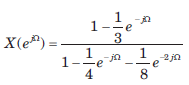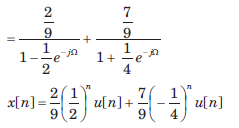Courses

# The Continuous - Time Fourier Series And The Discrete -Time Fourier Transform - MCQ Test

## 15 Questions MCQ Test Mock Tests Electronics & Communication Engineering GATE 2020 | The Continuous - Time Fourier Series And The Discrete -Time Fourier Transform - MCQ Test

Description
This mock test of The Continuous - Time Fourier Series And The Discrete -Time Fourier Transform - MCQ Test for Railways helps you for every Railways entrance exam. This contains 15 Multiple Choice Questions for Railways The Continuous - Time Fourier Series And The Discrete -Time Fourier Transform - MCQ Test (mcq) to study with solutions a complete question bank. The solved questions answers in this The Continuous - Time Fourier Series And The Discrete -Time Fourier Transform - MCQ Test quiz give you a good mix of easy questions and tough questions. Railways students definitely take this The Continuous - Time Fourier Series And The Discrete -Time Fourier Transform - MCQ Test exercise for a better result in the exam. You can find other The Continuous - Time Fourier Series And The Discrete -Time Fourier Transform - MCQ Test extra questions, long questions & short questions for Railways on EduRev as well by searching above.
QUESTION: 1

### Determine the Fourier series coefficient for given periodic signal x(t). Que: x(t) as shown in fig.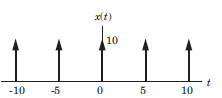​

Solution: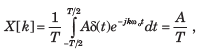A = 10 , T = 5, X [k] = 2

QUESTION: 2

### Determine the Fourier series coefficient for given periodic signal x(t). Que: x(t) as shown in fig.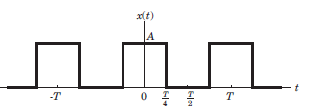​ ​ ​

Solution: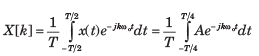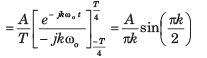QUESTION: 3

### Determine the Fourier series coefficient for given periodic signal x(t). Que: x(t) as shown in fig.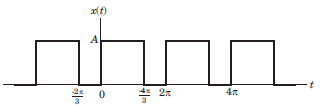​ ​ ​

Solution: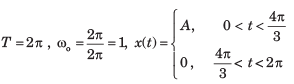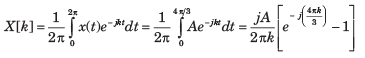QUESTION: 4

Determine the Fourier series coefficient for given periodic signal x(t).

Que: x(t) as shown in fig.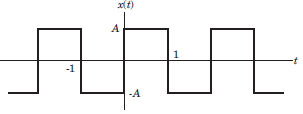​ ​ ​

Solution: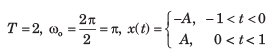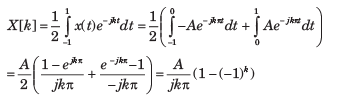QUESTION: 5

Determine the Fourier series coefficient for given periodic signal x(t).

x(t) = sin2t

Solution: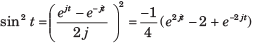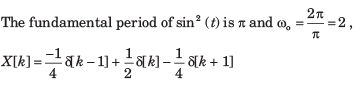QUESTION: 6

In the question, the FS coefficient of time-domain signal have been given. Determine the corresponding time domain signal and choose correct option.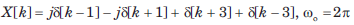Solution: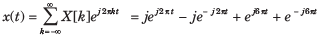= 2(cos 6πt - sin 2πt)

QUESTION: 7

In the question, the FS coefficient of time-domain signal have been given. Determine the corresponding time domain signal and choose correct option.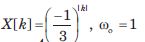Solution: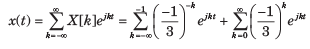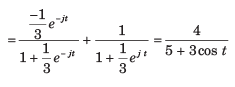QUESTION: 8

In the question, the FS coefficient of time-domain signal have been given. Determine the corresponding time domain signal and choose correct option.

Que: X[k] as shown in fig, wo  = π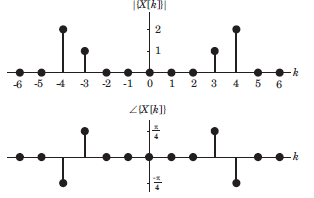Solution: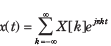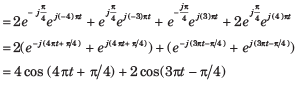QUESTION: 9

In the question, the FS coefficient of time-domain signal have been given. Determine the corresponding time domain signal and choose correct option.

Que: X[k] As shown in fig. , ωo = 2π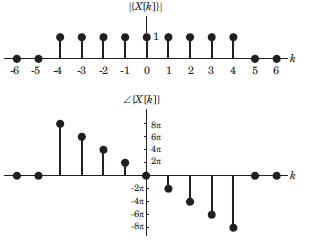Solution: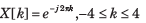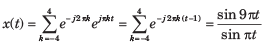QUESTION: 10

In the question, the FS coefficient of time-domain signal have been given. Determine the corresponding time domain signal and choose correct option.

Que: X[k] As shown in fig. , ωo = π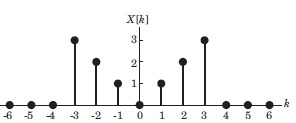Solution: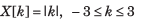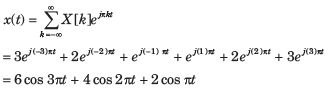QUESTION: 11

Consider a continuous time periodic signal x(t) with fundamental period T and Fourier series coefficients X[k]. Determine the Fourier series coefficient of the signal y(t) given in question and choose correct option.

Que: y(t) = x(t - t0 ) + x (t - t0 )

Solution:

x(t - t0) is also periodic with T.  TheFourier series coefficients X1[k] of x(t - t0) are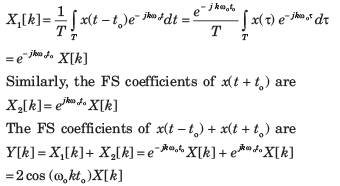QUESTION: 12

Consider a continuous time periodic signal x(t) with fundamental period T and Fourier series coefficients X[k]. Determine the Fourier series coefficient of the signal y(t) given in question and choose correct option.

Que: y(t) = Ev{x(t)}

Solution: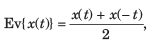The FS coefficients of x(t) are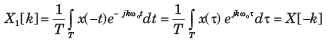Therefore, the FS coefficients of Ev{ x(t)} areQUESTION: 13

Consider a continuous time periodic signal x(t) with fundamental period T and Fourier series coefficients X[k]. Determine the Fourier series coefficient of the signal y(t) given in question and choose correct option.

Que: y(t) = Re{x(t)}

Solution: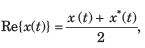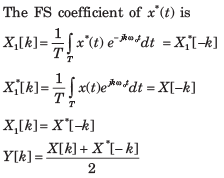QUESTION: 14

Determine the signal having the Fourier transform given in question.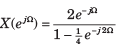Solution: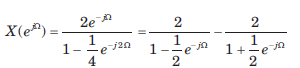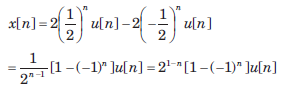QUESTION: 15

Determine the signal having the Fourier transform given in question.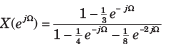Solution: